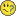QlikView Creating Analytics

Discussion Board for collaboration related to Creating Analytics for QlikView.

Announcements
QlikWorld 2020: Join us May 11 - 14, 2020 in Phoenix, AZ. Register early and save \$400. Learn More
Highlighted
New Contributor III

Nested set analysis?

I want to do some analysis that counts the number of items sold to customers with a total spend between certain spend thresholds.

E.g. Customers spending between 0-999 bought a total of 500 boxes of product A, 399 boxes of product B. Customers spending between 1000-1499 bought 300 boxes of product B etc.

My understanding is that I need to do a set analysis expression for each threshold spend whilst using product as the dimension.

I've got as far as writing an expression that looks like this:

sum({\$<location_id=P({<sum(threshold_value)={">0<1000"}>})threshold_quantity)

I've done something wrong here but I'm not sure what!With your help what I'm hoping to produce is a simple table that shows all of our hundreds of products and a count of units sold by the various thresholds.

Thanks for your help6 RepliesMVP

Re: Nested set analysis?

Not sure if I understand, could you maybe upload a small sample QVW?

I was thinking about something like this (in a chart with dimension Product):

=Count( {<Customer = {"=Sum(SpentValue) >= 0 and Sum(SpentValue) <1000"} >} DISTINCT BoxID)MVP

Re: Nested set analysis?

 sum({\$0<1000"}>})threshold_quantity) I've done something wrong here but I'm not sure what!

To me this looked like an implicit question, so I'll try to answer it.

Set modifiers (everything you put between < and > in a - in your case "nested" - set specification) change the data set for an expression by temporarily assigning other values to one or more listed fields. As a consequence, the equal sign in the middle is an assignment operator, not a comparison operator.

A second consequence of this statement is that the left hand side of the assignment operator should always be a field name. You cannot assign values larger than 0 and smaller than 1000 to the sum of all threshold_value values...

Best,

Peter

New Contributor III

Re: Nested set analysis?

Thanks for your reply. I haven't got a small enough QVW to upload.

The objective I'm trying to reach is a table that would have products listed down the left and columns that show each product's volumes by spend grouping. I wouldn't want to include the product itself within the expression as it's the dimension.

In plain english this is what I want to get:

How many boxes/units of each product we sell is purchased by customers spending between a and b, b and c, d and e.

There is nothing in our data that currently groups customers by spend and so it would need to be done in the set analysis (unless there's another way?).MVP

Re: Nested set analysis?

My intention was to do this by creating a field modifier for Customer with an advanced search expression

=Count( {<Customer = {"=Sum(SpentValue) >= 0 and Sum(SpentValue) <1000"} >} DISTINCT BoxID)

You can add more expressions to select customers with different spend amount:

=Count( {<Customer = {"=Sum(SpentValue) >= 1000 and Sum(SpentValue) <2000"} >} DISTINCT BoxID)

=Count( {<Customer = {"=Sum(SpentValue) >= 2000 and Sum(SpentValue) <5000"} >} DISTINCT BoxID)

You can also use variables in the expressions:

=Count( {<Customer = {"=Sum(SpentValue) >= a and Sum(SpentValue) <b"} >} DISTINCT BoxID)

=Count( {<Customer = {"=Sum(SpentValue) >= b and Sum(SpentValue) <c"} >} DISTINCT BoxID)

=Count( {<Customer = {"=Sum(SpentValue) >= c and Sum(SpentValue) <d"} >} DISTINCT BoxID)

New Contributor III

Re: Nested set analysis?

I've tried this as an example expression:

=sum({<location_id={"=sum(threshold_value)>1000 and sum(threshold_value)<1500"}>}threshold_quantity)

With the dimension as the SKU code of the products. There is some flaw with this though as when I add a second dimension which is the product description (a text field that describes the product) multiple descriptions are being assigned to each of the SKU codes when it should be 1 code to 1 description. So something can't be quite right?MVP

Re: Nested set analysis?

Hard to say without knowing your data model. Could you upload a small sample QVW?